# 2nd PUC Maths Question Bank Chapter 3 Matrices Ex 3.4

Students can Download Maths Chapter 3 Matrices Ex 3.4 Questions and Answers, Notes Pdf, 2nd PUC Maths Question Bank with Answers helps you to revise the complete Karnataka State Board Syllabus and score more marks in your examinations.

## Karnataka 2nd PUC Maths Question Bank Chapter 3 Matrices Ex 3.4

### 2nd PUC Maths Matrices NCERT Text Book Questions and Answers Ex 3.4

Question 1.
Using elementary transformations, find the inverse of each of the matrices, if it exists
in Exercise 1 to 17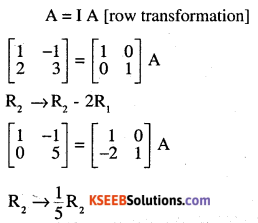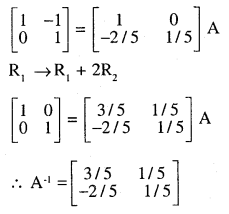Question 2.
$$\left[\begin{array}{ll}{2} & {1} \\{1} & {1}\end{array}\right]$$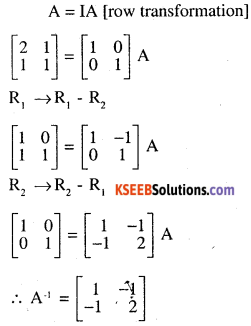Question 3.
$$\left[\begin{array}{ll}{1} & {3} \\{2} & {7}\end{array}\right]$$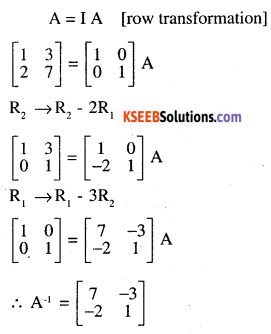Question 4.
$$\left[\begin{array}{ll}{2} & {3} \\{5} & {7}\end{array}\right]$$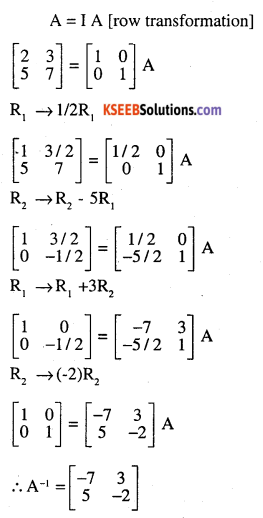Question 5.
$$\left[\begin{array}{ll}{2} & {1} \\{7} & {4}\end{array}\right]$$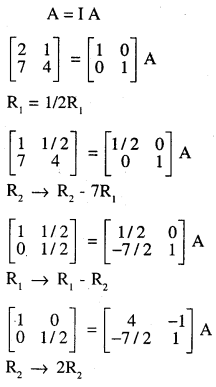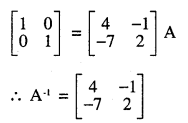Question 6.
$$\left[\begin{array}{ll}{2} & {5} \\{1} & {3}\end{array}\right]$$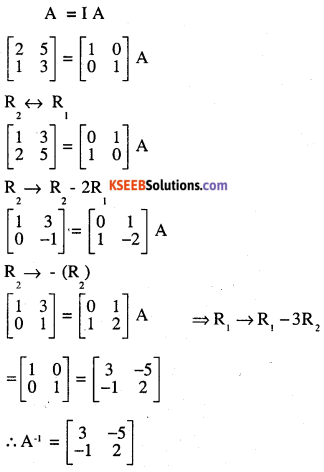Question 7.
$$\left[\begin{array}{ll}{3} & {1} \\{5} & {2}\end{array}\right]$$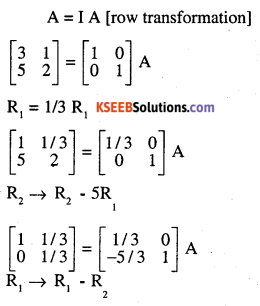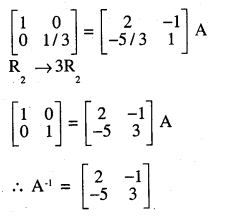Question 8.
$$\left[\begin{array}{ll}{4} & {5} \\{3} & {4}\end{array}\right]$$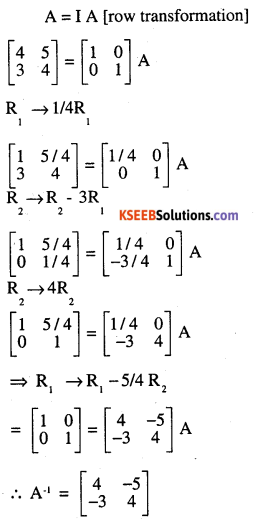Question 9.
$$\left[\begin{array}{rr}{3} & {10} \\{2} & {7}\end{array}\right]$$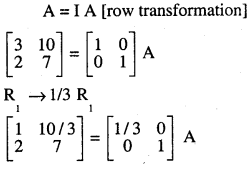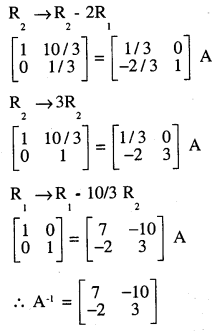Question 10.
$$\left[\begin{array}{cc}{3} & {-1} \\{-4} & {2}\end{array}\right]$$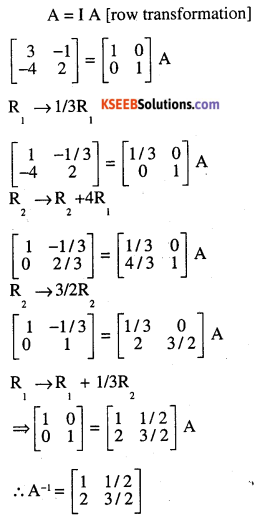Question 11.
$$\left[\begin{array}{ll}{2} & {-6} \\{1} & {-2}\end{array}\right]$$
A = I A[row transformation]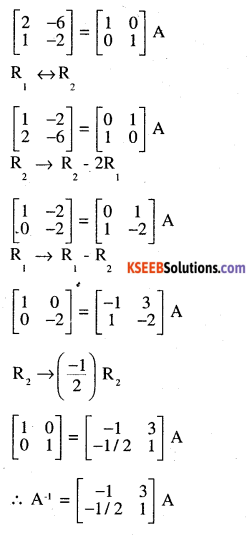Question 12.
$$\left[\begin{array}{rr}{6} & {-3} \\{-2} & {1}\end{array}\right]$$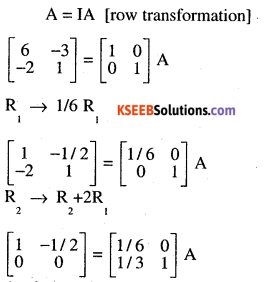As the matrix on LHS contain 0 only in the second row the given matrix is non-invertible. i.e. A 1 does not exist.Question 13.
$$\left[\begin{array}{cc}{2} & {-3} \\{-1} & {2}\end{array}\right]$$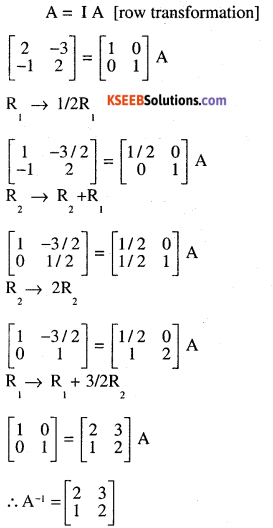Question 14.
$$\left[\begin{array}{ll}{2} & {1} \\{4} & {2}\end{array}\right]$$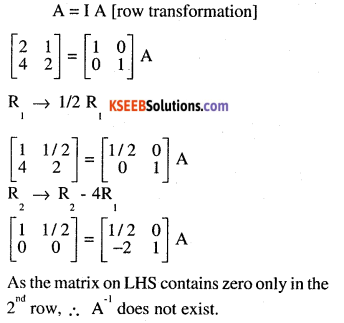Question 15.
$$\left[\begin{array}{ccc}{2} & {-3} & {3} \\{2} & {2} & {3} \\{3} & {-2} &{2}\end{array}\right]$$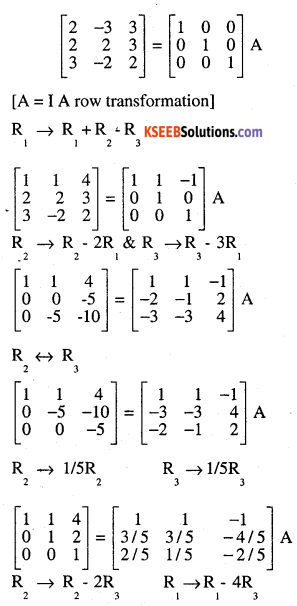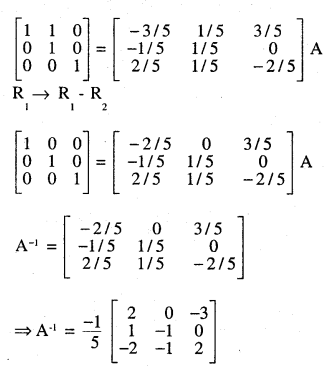Question 16.
$$\left[\begin{array}{rrr}{1} & {3} & {-2} \\{-3} & {0} & {-5} \\{2} & {5} & {0}\end{array}\right]$$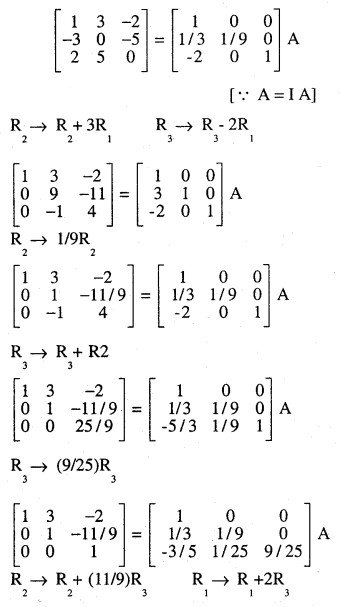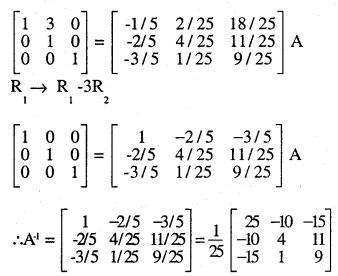Question 17.
$$\left[\begin{array}{rrr}{2} & {0} & {-1} \\{5} & {1} & {0} \\{0} & {1} & {3}\end{array}\right]$$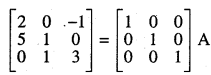Question 18.
Matrices A and B if inverse of each other only W
(A) AB = BA
(B) AB = BA = 0
(C) AB = 0,RA = I
(D) AB = BA = I